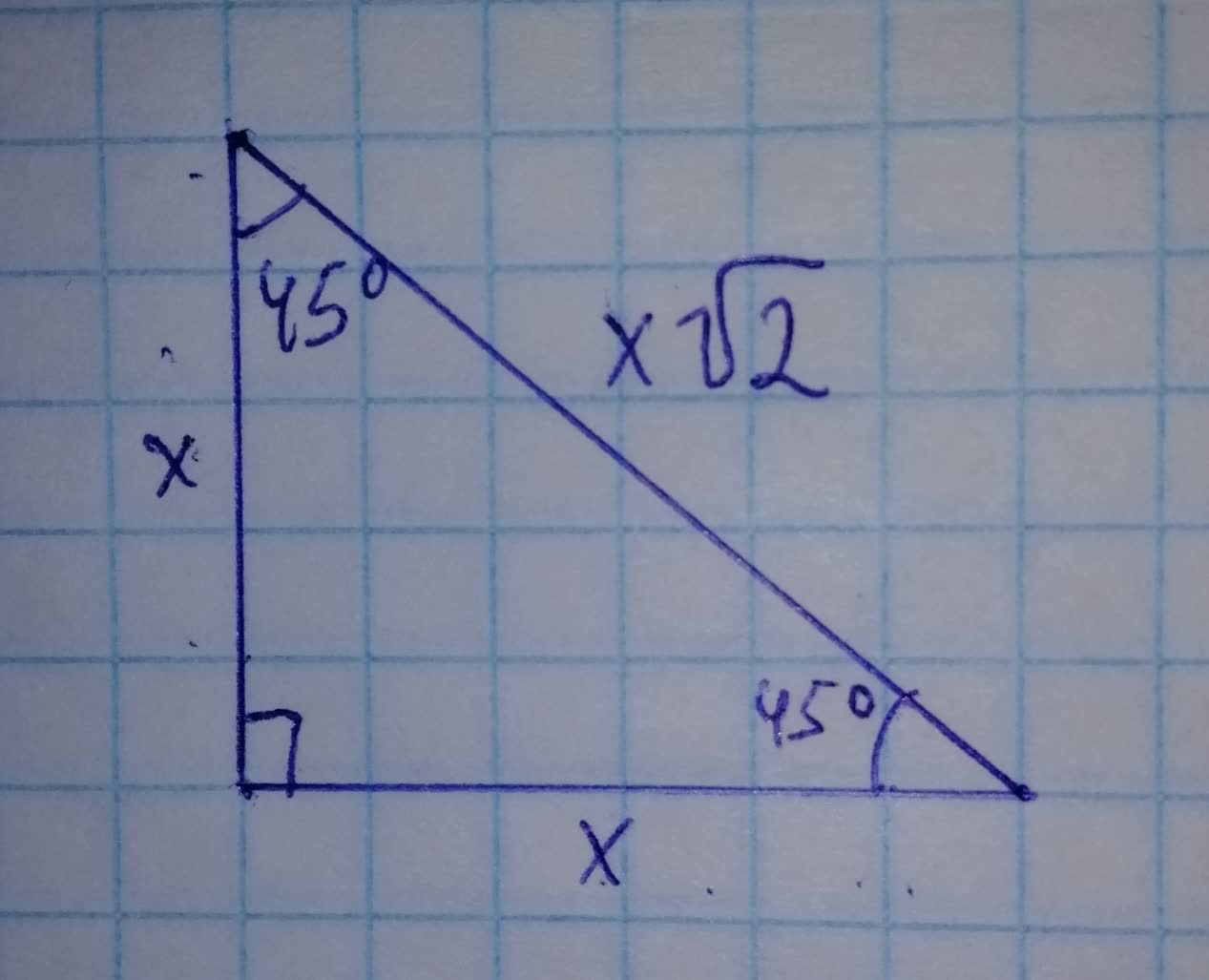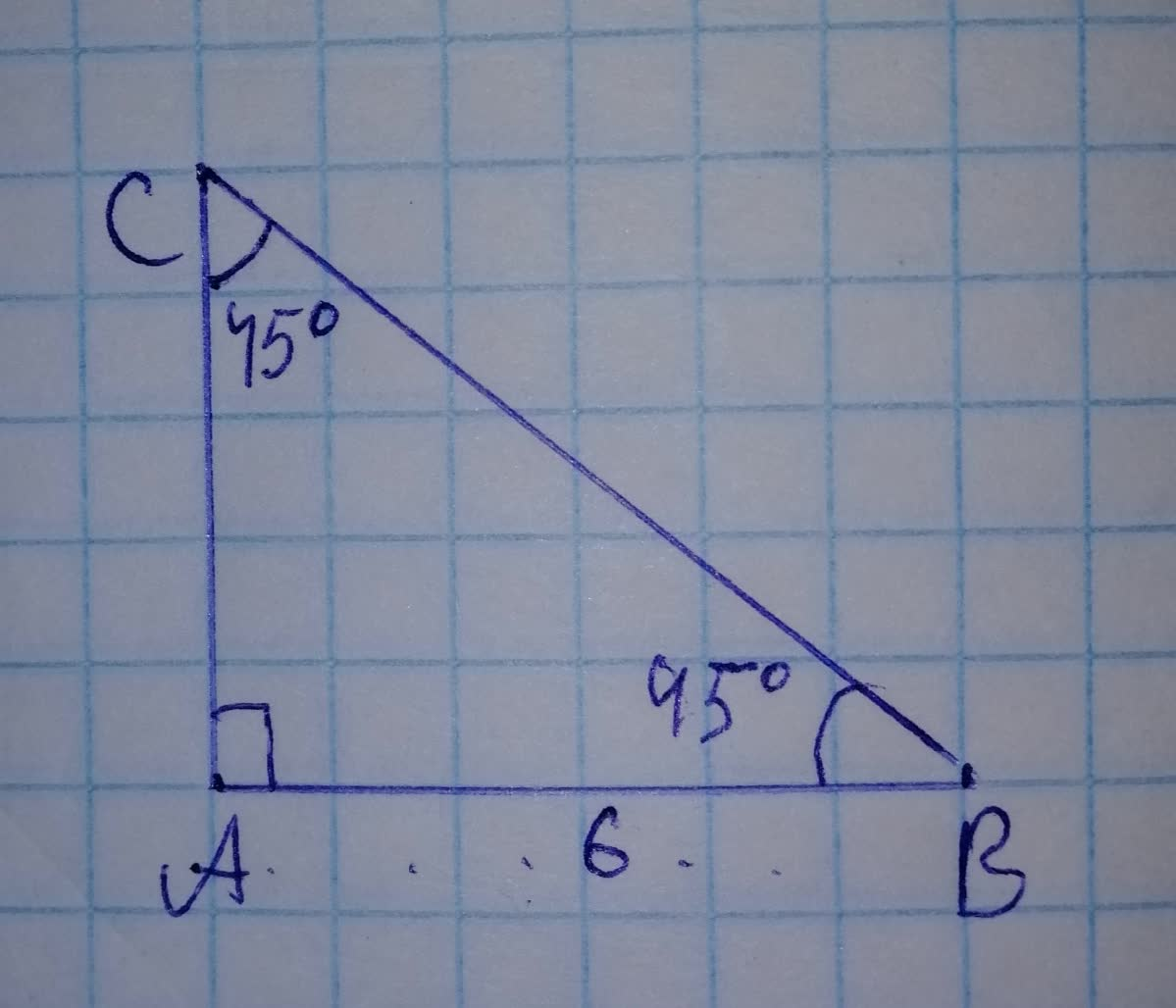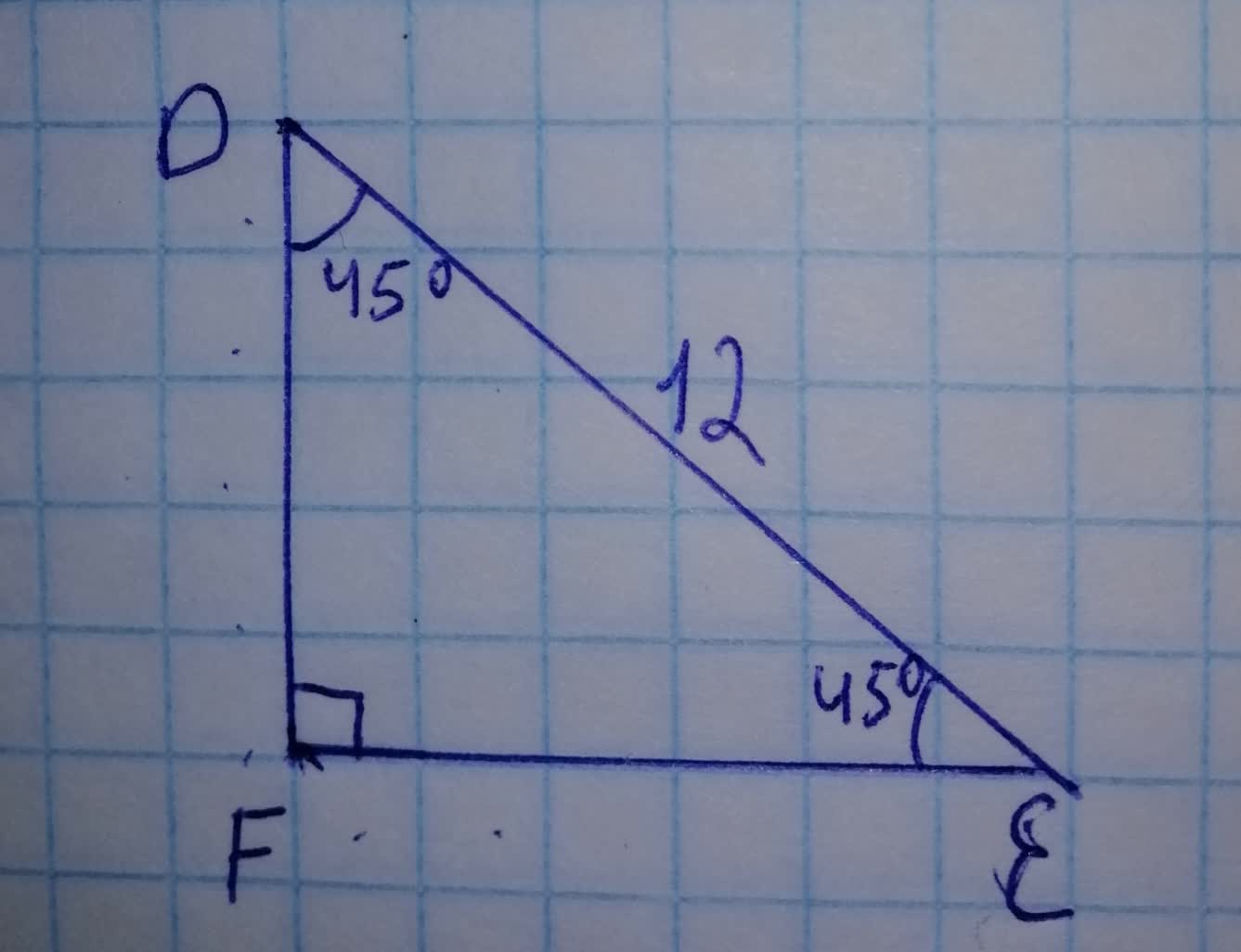# What do all these triangles have in common? a) 19610800081.jpg b) 19610800082.jpg c)gabioskay7 2021-11-22 Answered
What do all these triangles have in common?
a)b)c)• Questions are typically answered in as fast as 30 minutes

### Plainmath recommends

• Get a detailed answer even on the hardest topics.
• Ask an expert for a step-by-step guidance to learn to do it yourself.Momp1989
Step 1
As we can see all the three triangles in the image have their angles same as other
i.e. all the triangles have $$\displaystyle{45}^{{\circ}},\ {45}^{{\circ}},\ {90}^{{\circ}}$$ angles
this is also called isosceles right triangle
Step 2
A $$\displaystyle{45}^{{\circ}},{45}^{{\circ}},\ {90}^{{\circ}}$$ triangle is a special type of isosceles right triangle where the two legs are congruent to one another and the non-right angles are both equal to $$\displaystyle{45}^{{\circ}}$$ degrees. Many times, we can use the Pythagorean theorem to find the missing legs or hypotenuse of $$\displaystyle{45}^{{\circ}},\ {45}^{{\circ}},\ {90}^{{\circ}}$$ triangles. The ratio of the sides to the hypotenuse is always $$\displaystyle{1}:{1}:\sqrt{{{2}}}$$